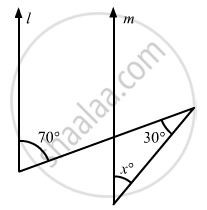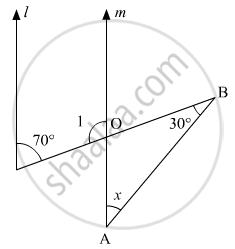# In the Given Figure, If Lines L and M Are Parallel Lines, Then X = - Mathematics

MCQ

In the given figure, if lines l and m are parallel lines, then x =• 70°

• 100°

• 40°

• 30°

#### Solution

We have the following figure:It is given that l || m

We know that consecutive interior angles are supplementary.

Therefore,

∠1 + 70° = 180°

∠1 = 180° - 70°

∠1 = 110°                (1)

$\angle1 = \angle AOB = 110 (\text { vertically opposite angles })$

In a triangle, we know that, the sum of the angles is supplementary.

In ΔAOB:

$30^\circ+ x + 110^\circ= 180^\circ$

$\Rightarrow x = 180 - 110 - 30 = 40$

Hence, the value of x will be $40^\circ$

Concept: Parallel Lines and a Transversal
Is there an error in this question or solution?

#### APPEARS IN

RD Sharma Mathematics for Class 9
Chapter 10 Lines and Angles
Q 22 | Page 56